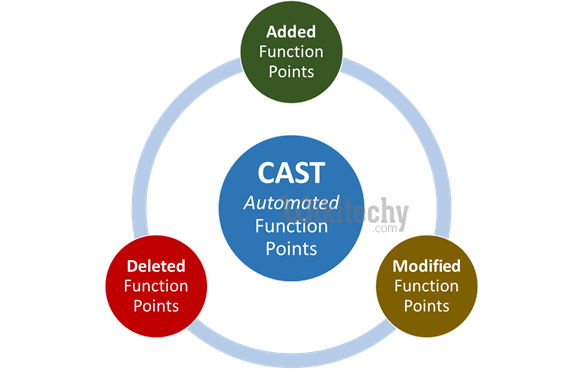# CAST Function in SQL - sql - sql tutorial - learn sql• In SQL Server (Transact-SQL), the CAST function converts an expression from one datatype to another datatype.
• The CAST function in SQL converts data from one data type to another.
• For example, we can use the CAST function to convert numeric data into character string data.

## Syntax

• The syntax of the CAST function is as follows:
• where [data type] is a valid data type in the RDBMS you are working with.

## Examples

• We use the following table for our examples.

## Table Student_Score

Column Name Data Type
StudentID integer
First_Name char(20)
Score float

This table contains the following rows:

## Table Student_Score

StudentID First_Name Score
1 Jenny 85.2
2 Bob 92.5
3 Alice 90
4 James 120.1

## Result:

First_Name Int_Score
Jenny 85
Bob 92
Alice 90
James 120
• In Example 1, we use the CAST function to convert the Score column from type FLOAT to INTEGER.
• When we do this, different RDMBS have different rules on how to handle the numbers after the decimal point.
• In the above example, the numbers after the decimal point are always truncated.

## Result:

First_Name Char_Score
Jenny 85.
Bob 92.
Alice 90
James 120
• In Example 2, we use the CAST function to convert the SCORE column from type FLOAT to CHAR(3).
• When we do this, we only take the first three characters.
• So, if there are more than three characters, everything after the first three characters is discarded.